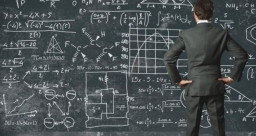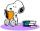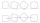# Equation with one variable

Solve the following equation with one unknown:

5(7s + 5) =130

s =  3

### Step-by-step explanation:

5(7s + 5) =130

5•(7•s + 5) =130

35s = 105

s = 3

Our simple equation calculator calculates it.Did you find an error or inaccuracy? Feel free to write us. Thank you!Tips to related online calculators
Do you have a linear equation or system of equations and looking for its solution? Or do you have a quadratic equation?

## Related math problems and questions:

• Eq1Solve equation: 4(a-3)=3(2a-5)
• Find xSolve: if 2(x-1)=14, then x= (solve an equation with one unknown)
• Unknown number xyzFind the number that its triple is 24. Solve by equation.
• Equation algebraogramSolve the equation: oco + ivo = cita How much has the task of solutions?
• NumberDetermine unknown number if you know that difference between five times and triple of number is 42.
• Unknown numberI think the number: Its half is 16 bigger than his quarter. I think the number N . ..Why does 1 3/4 + 2 9/10 equal 4.65? How do you solve this?
• Find unknown 2Find unknown denominator: 2/3 -5/? = 1/4
• Unknown xIf we add to unknown number 21, then divide by 6 and then subtract 51, we get back an unknown number. What is this unknown number?
• Unknown number 24I think the number: a - is the same as the square area that has the 12th circumference. What is this number? b - its half is 7 times bigger than its quarter. Is this the number?
• Perimeter to area 2Find the area of a square whose perimeter is 100 cm with solve.
• If-then equationIf 5x - 17 = -x + 7, then x =
• Simple equationFind the value of x in 21-3x=x+1
• Do you solve this?Determine area S of rectangle and length of its sides if its perimeter is 102 cm.
• One-thirdA one-third of unknown number is equal to five times as great as the difference of the same unknown number and number 28. Determine the unknown number.
• Number unknownAdela thought the two-digit number, she added it to its ten times and got 407. What number does she think?
• Unknown numberFind the unknown number equal to a quarter of a fifth of a number, which is by 152 more than an unknown number.